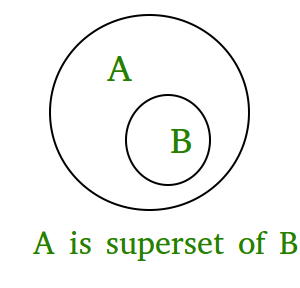Related Articles
issuperset() in Python
• Difficulty Level : Easy
• Last Updated : 05 Feb, 2018

The issuperset() method returns True if all elements of a set A occupies set B which is passed as an argument and returns false if all elements of B not present in A.
This means if A is a superset of B then it returns true; else False

Syntax:

```A.issuperset(B)
checks whether A is a superset of B or not.```

Returns:

`True if A is a superset of B; otherwise false.`Code 1:

 `# Python program to demonstrate working of ` `# issuperset(). ` ` `  `A ``=` `{``4``, ``1``, ``3``, ``5``} ` `B ``=` `{``6``, ``0``, ``4``, ``1``, ``5``, ``0``, ``3``, ``5``} ` ` `  `print``(``"A.issuperset(B) : "``, A.issuperset(B)) ` ` `  `# B is superset of A ` `print``(``"B.issuperset(A) : "``, B.issuperset(A)) `

Output:

```A.issuperset(B) :  False
B.issuperset(A) :  True
```

Code 2:

 `# Python program to demonstrate working  ` `# of issuperset(). ` ` `  `A ``=` `{``1``, ``2``, ``3``} ` `B ``=` `{``1``, ``2``, ``3``, ``4``, ``5``} ` `C ``=` `{``1``, ``2``, ``4``, ``5``} ` ` `  `print``(``"A.issuperset(B) : "``, A.issuperset(B)) ` ` `  `print``(``"B.issuperset(A) : "``, B.issuperset(A)) ` ` `  `print``(``"A.issuperset(C) : "``, A.issuperset(C)) ` ` `  `print``(``"C.issuperset(B) : "``, C.issuperset(B)) `

Output:

```A.issuperset(B) :  False
B.issuperset(A) :  True
A.issuperset(C) :  False
C.issuperset(B) :  False```

Attention geek! Strengthen your foundations with the Python Programming Foundation Course and learn the basics.

To begin with, your interview preparations Enhance your Data Structures concepts with the Python DS Course.

My Personal Notes arrow_drop_up
Recommended Articles
Page :